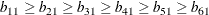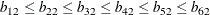### Example 29.32 Ordinal Relations among Factor Loadings

The same data set as in Example 29.31 is used in McDonald (1980) for analysis with ordinally constrained factor loadings. In Example 29.27, the results of the linearly constrained factor analysis show that the loadings of the two factors are ordered as 2, 1, 3, 4, 6, 5. McDonald (1980) then tests the hypothesis that the factor loadings are all nonnegative and can be ordered in the following manner:In this example, you implement these ordinal relationships by using the LINCON statement in the following COSAN model specification:

```proc calis data=Kinzer nobs=326 nose;
cosan
var= var1-var6,
D(6,DIA) * B(2,GEN) + D(6,DIA) * Psi(6,DIA);
matrix B
[ ,1]= b11 b21 b31 b41 b51 b61,
[ ,2]= 0.  b22 b32 b42 b52 b62;
matrix Psi
[1,1]= psi1-psi6;
matrix D
[1,1]= d1-d6 ;
lincon
b61  <= b51,
b51  <= b41,
b41  <= b31,
b31  <= b21,
b21  <= b11,
0.  <= b22,
b22  <= b32,
b32  <= b42,
b42  <= b52,
b52  <= b62;

/* SAS Programming Statements */
/* 6 Constraints on Correlation structures */
psi1 = 1. - b11 * b11;
psi2 = 1. - b21 * b21 - b22 * b22;
psi3 = 1. - b31 * b31 - b32 * b32;
psi4 = 1. - b41 * b41 - b42 * b42;
psi5 = 1. - b51 * b51 - b52 * b52;
psi6 = 1. - b61 * b61 - b62 * b62;
vnames
B   = [factor1 factor2],
Psi = [var1-var6],
D   = Psi;
run;
```

As in Example 29.31, correlation structures are analyzed in the current example so that the unique variance parameters `psi1``psi6` are defined as functions of the loadings in the SAS programming statements. However, the loading parameters are no longer not constrained in the current model. Instead, you impose ordinal constraints on the loading parameters. First, `b21` is fixed at 0 for identification purposes. Then, you use the LINCON statement to specify the ordinal relations of the factor loadings.

As shown in Output 29.32.1, the solution converges in 12 iterations. In the fit summary table, the chi-square test statistic is 8.48 (df = 6, p = 0.20). This indicates a good fit. However, in the model there are 11 loading parameters (the `b`’s) and 6 population standard deviation parameters (the `d`’s). The degrees of freedom should have been 4 = 21 – 11 – 6, but why is this number 6 in the fit summary table?

Output 29.32.1: Final Iteration Status and Fit

Optimization Results
Iterations 12 Function Calls 29
Jacobian Calls 14 Active Constraints 2
Objective Function 0.0260990149 Max Abs Gradient Element 2.7626747E-6
Lambda 0 Actual Over Pred Change 1.1572072766
Radius 0.0000851592

 Convergence criterion (ABSGCONV=0.00001) satisfied.

Fit Summary
Chi-Square 8.4822
Chi-Square DF 6
Pr > Chi-Square 0.2049

The reason is that there are two active constraints in the solution, resulting in two free parameters fewer in the final solution than originally specified. Active constraints are those inequality constraints that are fulfilled on the boundary equalities. As shown in the Optimization Results table, the number of active constraints for the current fitting is two. The default treatment in PROC CALIS is to treat these active constraints as if they were going to happen for all possible repeated sampling. This might as well be seen as fitting the active equality constraints on every possible repeated sample. This results in an increase of the degrees of freedom for model fit, as adjusted in the current fit summary table in Output 29.32.1. To warn you about the degrees-of-freedom adjustment, the following messages are also printed with the output:

```WARNING: There are 2 active boundary or linear inequality constraints at
the solution. The standard errors and chi-square test statistic
assume that the solution is located in the interior of the
parameter space; hence, they do not apply if it is likely that
some different set of inequality a constraints could be active.

NOTE: The degrees of freedom are increased by the number of active
constraints.  The number of parameters in calculating fit indices
is decreased by the number of active constraints. To turn off the
adjustment, use the NOADJDF option.
```

When active constraints are encountered, you need to be cautious about two implications. First, the estimates fall on the boundary of the parameter space originally specified. As shown in Output 29.32.2, estimates for `b11` and `b21` are the same, and so are the pair of estimates for `b52` and `b62`. These pairs of parameters were originally constrained by inequalities in the model. For example, `b62` was constrained to be at least as large as `b52`. The fact that this constraint is honored only on the bound means that a better model fit might exist with `b62` being smaller than `b52`. Similarly, a better model fit might result without requiring `b11` to be at least as large as `b21`. Therefore, solutions with active boundary constraints might imply that the original strict inequality constraints are not appropriate for the data.

Output 29.32.2: Estimation of the Factor Loading Matrix B

Model Matrix B
(6 x 2 General Rectangular Matrix)
factor1 factor2
var1
 0.7100 [b11]
 0
var2
 0.7100 [b21]
 0.0393 [b22]
var3
 0.6799 [b31]
 0.2463 [b32]
var4
 0.6561 [b41]
 0.3295 [b42]
var5
 0.5541 [b51]
 0.5432 [b52]
var6
 0.4733 [b61]
 0.5432 [b62]

Output 29.32.3: Estimation of the Scaling Matrix D and Unique Covariance Matrix Psi

Model Matrix D
(6 x 6 Diagonal Matrix)
var1 var2 var3 var4 var5 var6
var1
 1.0022 [d1]
 0
 0
 0
 0
 0
var2
 0
 0.9985 [d2]
 0
 0
 0
 0
var3
 0
 0
 1.0004 [d3]
 0
 0
 0
var4
 0
 0
 0
 1.0004 [d4]
 0
 0
var5
 0
 0
 0
 0
 0.9990 [d5]
 0
var6
 0
 0
 0
 0
 0
 1.0021 [d6]

Model Matrix Psi
(6 x 6 Diagonal Matrix)
var1 var2 var3 var4 var5 var6
var1
 0.4959 [psi1]
 0
 0
 0
 0
 0
var2
 0
 0.4944 [psi2]
 0
 0
 0
 0
var3
 0
 0
 0.4771 [psi3]
 0
 0
 0
var4
 0
 0
 0
 0.4610 [psi4]
 0
 0
var5
 0
 0
 0
 0
 0.3979 [psi5]
 0
var6
 0
 0
 0
 0
 0
 0.4809 [psi6]

The second implication for the presence of active constraints is that the chi-square test statistic and the standard error estimates are computed as if repeated samples were fitted by the model with the presence of the active equality constraints. The degrees-of-freedom adjustment by PROC CALIS is based on this assumption. However, if the particular active constraints reflect only a rare sampling event, the degrees-of-freedom adjustment (or even the computation of the chi-square statistic and standard error estimates) might not be justified. Unfortunately, whether the active constraints are reflecting the truth of the model or pure sampling fluctuation is usually difficult to determine.